# On “Stock correlation has been rising”

Ticker Sense posted about the mean correlation of the S&P 500. The plot there — similar to Figure 1 — shows that correlation has been on the rise after a low in February.

Figure 1: Mean 50-day rolling correlation of S&P 500 constituents to the index.For me, this post raised a whole lot more questions than answers.

## Which correlation?

When I think of correlation, I think of the correlation of the constituents among themselves.  But the post is of the correlation of each constituent with the index.  So, does it matter?

Figure 2: Comparison of correlations:  each constituent to the index (gold line) and constituents among themselves (blue line).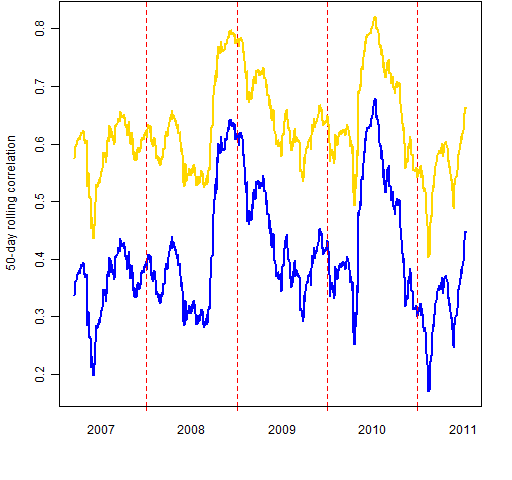Figure 2 seems to say that it probably doesn’t matter as long as you know which you are talking about.  However, if we look at the difference of the two methods — as in Figure 3 — we see something different.  It looks like perhaps something really has begun recently.

Figure 3: Constituent to index mean correlation minus mean intra-constituent correlation.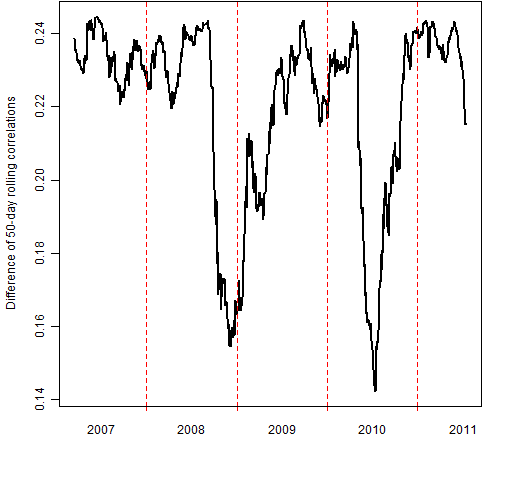## Correlation versus level

The Ticker Sense plot shows the index level along with the correlation — implying there is a relationship.  The relationship that we would care about is if correlation predicts returns.  Well, maybe.  I’m waiting to be convinced.

## Variability from the stocks

From now on, we’ll stay in the original playground of the correlation of each constituent to the index.

Figure 1 (and the original plot) is a solid line as if we actually knew the value at each point.  In actuality there are sources of variability that means we don’t really know where the line is.

One source of variability is the constituents.  We can explore how much variability they instill into the computation by using the statistical bootstrap.

Figure 4: Mean 50-day rolling correlation of S&P 500 constituents to the index (gold) plus 95% bootstrap confidence interval (purple).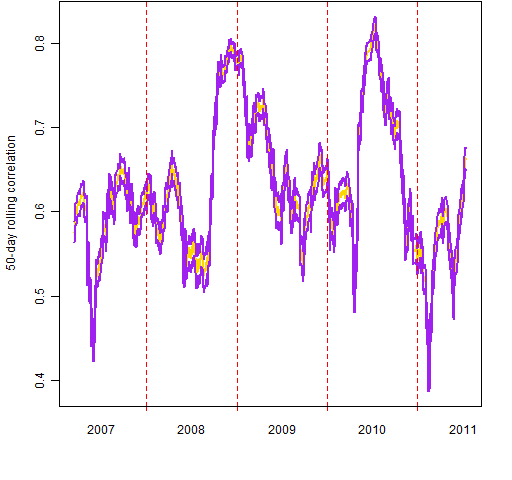Figure 4 shows the 95% confidence interval at each point from constituent variability.  The confidence interval is narrow relative to the moves over time.  As Figure 5 shows, the width of the confidence interval is not constant through time.

Figure 5: Width of the 95% bootstrap confidence interval of constituent to index correlation.## Variability within the window

There is another source of variability: we are estimating the correlations with only 50 observations.  Figure 6 shows the variability attributable to the finite sample for the most recent window (through 2011 July 15).

Figure 6: Distribution from bootstrapping over the last 50 days.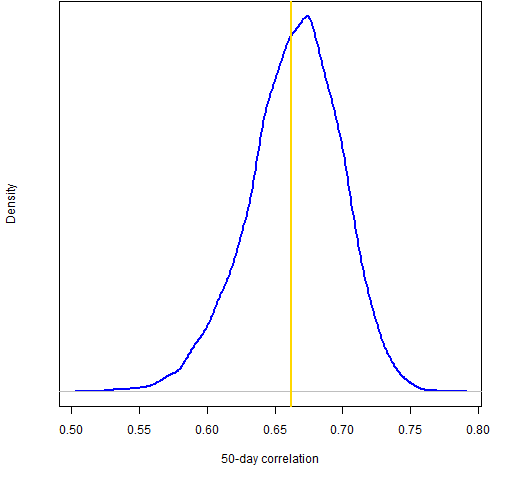So there is substantial variability here. Figure 7 adds the 95% confidence interval due to days for the final window to the time series view.

Figure 7: 95% confidence interval from bootstrapping the final  window (blue) along with the 95% confidence interval for bootstrapping constituents at each point.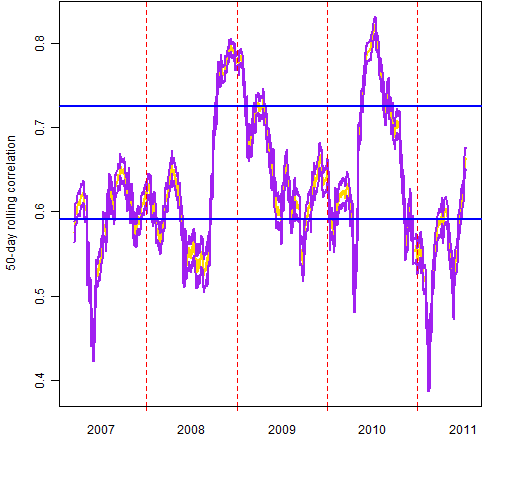We have a problem.  There is an uncertainty principle at work: if we widen the window to reduce variability, then we start to lose the dynamic nature of the correlation.  We can’t know both location and momentum.

## Similar post

Elevated stock correlations

## Appendix R

You can get a script of the R analysis and/or get a file of the R functions including those that create the figures.

This analysis — as does that of “Weight compared to risk fraction” —  starts off by using the QuantTrader blog post “Downloading S&P 500 data to R”. In particular the post includes a link to a file that contains the stock symbols for the constituents.

sp500.symbols <- scan(url(sp500.symbol.url), what="")

Whenever you get data, it should be standard practice to graphically inspect it.  Such an inspection paid off in this case: something went wrong with the recent data for ‘Q’ and the same price was repeated for several days.  That stock was eliminated from the analysis.  Better would be to investigate and fix the data.

Subscribe to the Portfolio Probe blog by Email

This entry was posted in Quant finance, R language and tagged , , , . Bookmark the permalink.

### 4 Responses to On “Stock correlation has been rising”

1.anon says:

when I stepped through your script, “Q” was 362, not 367.

2.Pat says:

Dear anon,

I’m not surprised. The reading of data from Yahoo! seems to be rather a random process. I suspect that I got quite a good run with 478 stocks that had the full data. My function that puts the data together throws out data that doesn’t have all the dates of the first stock read, so it is very strict. You could revise that function to make it less picky.